# Drawing and identifying a polygon in the coordinate plane Online Quiz

Following quiz provides Multiple Choice Questions (MCQs) related to Drawing and identifying a polygon in the coordinate plane. You will have to read all the given answers and click over the correct answer. If you are not sure about the answer then you can check the answer using Show Answer button. You can use Next Quiz button to check new set of questions in the quiz.Q 1 - Identify the polygon formed in the coordinate plane below.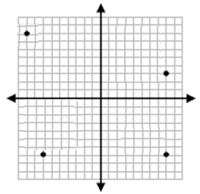### Explanation

Step 1:

There are four dots in the given coordinate plane.

Step 2:

Joining these dots with line segments, the polygon formed has four sides and is a Quadrilateral.Q 2 - Identify the polygon formed in the coordinate plane below.### Explanation

Step 1:

There are five dots in the given coordinate plane.

Step 2:

Joining these dots with line segments, the polygon formed has five sides and is a Pentagon.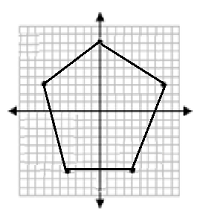Q 3 - Identify the polygon formed in the coordinate plane below.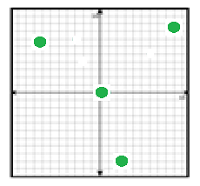### Explanation

Step 1:

There are four dots in the given coordinate plane.

Step 2:

Joining these dots with line segments, the polygon formed has four sides and is a QuadrilateralQ 4 - Identify the polygon formed in the coordinate plane below.### Explanation

Step 1:

There are four dots in the given coordinate plane.

Step 2:

Joining these dots with line segments, the polygon formed has four sides and is a Quadrilateral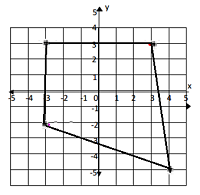Q 5 - Identify the polygon formed in the coordinate plane below.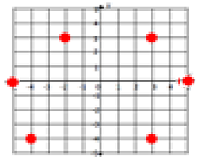### Explanation

Step 1:

There are six dots in the given coordinate plane.

Step 2:

Joining these dots with line segments, the polygon formed has six sides and is a Hexagon.Q 6 - Identify the polygon formed in the coordinate plane below.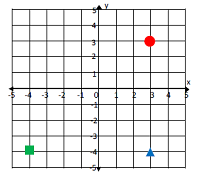### Explanation

Step 1:

There are three dots in the given coordinate plane.

Step 2:

Joining these dots with line segments, the polygon formed has three sides and is a TriangleQ 7 - Identify the polygon formed in the coordinate plane below.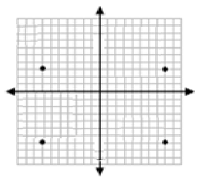### Explanation

Step 1:

There are four dots in the given coordinate plane.

Step 2:

Joining these dots with line segments, the polygon formed has four sides and is a Rectangle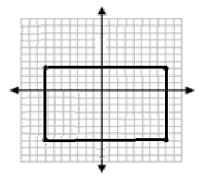Q 8 - Identify the polygon formed in the coordinate plane below.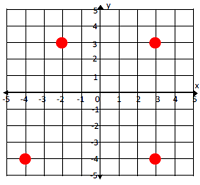### Explanation

Step 1:

There are four dots in the given coordinate plane.

Step 2:

Joining these dots with line segments, the polygon formed has four sides and is a QuadrilateralQ 9 - Identify the polygon formed in the coordinate plane below.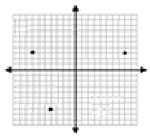### Explanation

Step 1:

There are three dots in the given coordinate plane.

Step 2:

Joining these dots with line segments, the polygon formed has three sides and is a TriangleQ 10 - Identify the polygon formed in the coordinate plane below.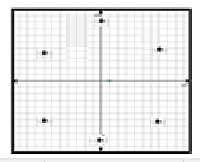### Explanation

Step 1:

There are six dots in the given coordinate plane.

Step 2:

Joining these dots with line segments, the polygon formed has six sides and is a Hexagondrawing_and_identifying_polygon_in_coordinate_plane.htm